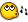# Pixelsearch Combined With Mouse Coords?

## Recommended Posts

NOTE: I figured out how exactly to use Pixel commands/functions better by studying some other scripts and looking at the help files, but now i'm having difficulty with basic functionality (ie: The given application does not accept the Send("{DOWN}) command, and i do not know how to access the mouse wheel through AU scripts)

What i am trying to do seems like it would be viable within the AutoIt realm of functionality, but i can't figure out exactly how i would go about doing it.

I'm trying to add something to a script of mine, that finds if a certain pixel combination appears in a pre-chosen area, and then clicks on that area.

I know that i can use this bit of script...

```; Setup our hotkey
HotKeySet("^!p", "GetPixel")

; Set up a low-CPU use infinite loop
While 1
Sleep(1000)
WEnd

Func GetPixel()
; Get the current mouse pointer co-ords
\$pos = MouseGetPos()

; Get the colour of the pixel currently under the pointer
\$col = PixelGetColor(\$pos, \$pos)

MsgBox(4096, "Pixel under mouse was:", \$col & " (0x" & Hex(\$col, 6) & ")" )

; Completely exit the script
Exit
EndFunc```

...to find out what (mouse coords and pixels) lies below the mouse pointer.

But, how can i get it to essentially scan a pre-chosen "box" of coords on the active window for certain pixels, find if they are there, click on them, if not, execute another function?

Ex.: The bot scrolls down a nav bar on a web page, and searches just in that nav bar for the word "Chevrolet". When it finds the word Chevrolet, no matter where it is, it clicks on that word. If it can't find the word, it scrolls again, then checks, then scrolls, until it finds it.

I hope this makes sense, big thanks to anyone that can help me out!Edited by tetris002
##### Share on other sites

I can't seem to find the original post, but josbe posted an excellent example of this:

```;// GetPixColor
;// Desc: Capture a color screen's and returns the values in Hexadecimal & RGB formats (for web).
;// By: JoSBE (mrjosbe@yahoo.com)
;// Especially for webmasters.;)
;// Free to modify and make better.;)
;// NOTE: The time to response depend to machine speed's. (SplashText)

If StringReplace(@AutoItVersion, ".", "") < 3085 then
msgbox(0, "Error", "Some functions in this script aren't available for your installed version," & @LF & "Please install the latest version of AutoIt3.")
exit
EndIf

;// You can end the process pressing the keys stored in "\$ks".
\$ks= "^+Q"
\$End= 1
HotKeySet(\$ks, "ELoop")
\$width_splash= 120
\$height_splash= 30

;// A remainder
msgbox(64, "Remember", "To end, press: " & VKEYS(\$ks))

while \$END == 1
\$pix = MouseGetPos()
\$var = PixelGetColor( \$pix, \$pix)
\$hex= hex(\$var, 6)
;// Arranging the AutoIt output
\$hexarr= stringright(\$hex, 2) & stringmid(\$hex, 3, 2) & stringleft(\$hex, 2)

;// Intelligent position, move the splash if not visible in Screen
If \$pix < @desktopwidth - \$width_splash Then
\$posX= \$pix+8
Else
\$posX= \$pix - \$width_splash - 8
EndIf
If \$pix < @desktopheight - \$height_splash Then
\$posY= \$pix+8
Else
\$posY= \$pix - \$height_splash - 8
EndIf
SplashTextOn("", "HEX= " & Chr(34) & "#"& \$hexarr & Chr(34) & @LF & "RGB= " & RGB(\$hex), \$width_splash, \$height_splash, \$posX, \$posY, 1, "arial", "8", "")
wend

SplashOff()
;// Optional copy the values to clipboard
\$op= msgbox(4, "?", "Copy the values color's to clipboard?")
If \$op = 6 then ClipPut("HEX= " & Chr(34) & "#"& \$hexarr & Chr(34) & " | RGB=" & RGB(\$hex))
exit

;; SMALL FUNCTIONS
;¯'¯'¯'¯'¯'¯'¯'¯'¯'¯'¯'¯'¯'¯'¯'¯'¯'¯'¯'¯'¯'¯'¯'¯'¯'¯'¯'¯'¯'¯'¯'
;// This little ends the ''while'' loop
Func ELoop()
\$END= 0
EndFunc
;¯'¯'¯'¯'¯'¯'¯'¯'¯'¯'¯'¯'¯'¯'¯'¯'¯'¯'¯'¯'¯'¯'¯'¯'¯'¯'¯'¯'¯'¯'¯'
Func RGB(\$hex)
Local \$val
Local \$rgb
\$val= StringLower(\$hex)
Dim \$v

For \$i=0 to 6
\$v[\$i]= GDec(stringmid(\$val, \$i+1, 1))
Next

\$x= (\$v * 16) + \$v
\$y= (\$v * 16) + \$v
\$z= (\$v * 16) + \$v
;; arrange
\$rgb= "(" & \$z & ", " & \$y & ", " & \$x & ")"
Return \$rgb
EndFunc

;¯'¯'¯'¯'¯'¯'¯'¯'¯'¯'¯'¯'¯'¯'¯'¯'¯'¯'¯'¯'¯'¯'¯'¯'¯'¯'¯'¯'¯'¯'¯'
;// Convert HEX to DEC
Func GDec(\$hex)
Local \$n
Local \$hex
\$n= \$hex

Select
Case \$hex == "a"
\$n= 10
Case \$hex == "b"
\$n= 11
Case \$hex == "c"
\$n= 12
Case \$hex == "d"
\$n= 13
Case \$hex == "e"
\$n= 14
Case \$hex == "f"
\$n= 15
EndSelect
Return \$n
EndFunc

;¯'¯'¯'¯'¯'¯'¯'¯'¯'¯'¯'¯'¯'¯'¯'¯'¯'¯'¯'¯'¯'¯'¯'¯'¯'¯'¯'¯'¯'¯'¯'
;// Verify the keys and returns in text format.
Func VKEYS(\$kstring)
Local \$tx
Local \$out
Local \$fout
\$fout= ""
For \$i=1 to StringLen(\$kstring)
\$tx= StringMid(\$kstring, \$i, 1)
\$out = \$tx
If \$out == "!" then \$out= "ALT"
If \$out == "+" then \$out= "SHIFT"
If \$out == "^" then \$out= "CTRL"
If \$i <> StringLen(\$kstring) then
\$fout= \$fout & chr(34) & \$out & chr(34) & " + "
else
\$fout= \$fout & chr(34) & \$out & chr(34)
EndIF
Next
Return \$fout
EndFunc```

## Create an account or sign in to comment

You need to be a member in order to leave a comment

## Create an account

Sign up for a new account in our community. It's easy!

Register a new account

• ### Recently Browsing   0 members

×

• Wiki

• Back

• #### Beta

• Git
• FAQ
×
• Create New...